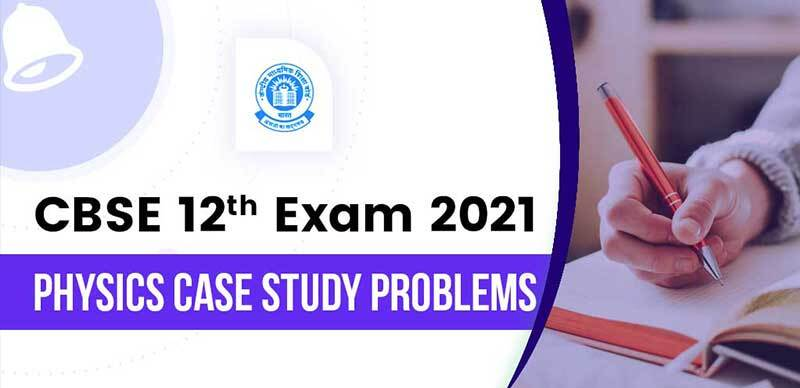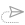# CBSE 12th Exam 2021 : Physics Case Study Problems

In Coming Exams, CBSE will ask two Case Study Questions in the CBSE class 12 Physics questions paper. Question numbers 15 and 16 are case-based questions where 5 MCQs will be asked based on a paragraph. Each theme will have five questions and students will have a choice to attempt any four of them.

Here are some example questions Based On Case Study Problems:

Question 1.

Read  the following source and answer any four out of the following questions:
Electric charge is the physical property of matter that causes it to experience a force when placed in an electromagnetic field. There are two types of charges positive and negative charges. Also, like charges repel each other whereas unlike charges attract each other.1. Charge on a body which carries 200 excess electrons is:
1. -3.2 × 10-18 C
2. 3.2 ×  10 18 C
3. -3.2 × 10-17 C
4. 3.2 × 10 -17  C
2. Charge on a body  which carries 10 excess electrons is:
1. -1.6 × 10-18 C
2. 1.6 × 10 -18 C
3. 2.6 × 10-18 C
4. 1.6 × 10-21 C
3. Mass of electron is:
1. 9.1 × 10-31 kg
2. 9.1 × 10-31 g
3. 1.6 × 10-19 kg
4. 1.6 × 10-19 g
4. A body is positively charged, it implies that:
1. there is only a positive charge in the body
2. there is positive as well as negative charge in the body but the positive charge is more than negative charge
3. there is equally positive and negative charge in the body but the positive charge lies in the outer regions
4. the negative charge is displaced from its position
5. On rubbing, when one body gets positively charged and other negatively charged, the electrons transferred from positively charged body to negatively charged body are:
1. valence electrons only
2. electrons of inner shells
3. both valence electrons and electrons of the inner shell.
4. none of the above

Question 2:

Read the following source and answer any four out of the following questions:
Resistance is a measure of the opposition to current flow in an electrical circuit. Resistance is measured in ohms. Also Resistivity, the electrical resistance of a conductor of unit cross-sectional area, and unit length. … A characteristic property of each material, resistivity is useful in comparing various materials on the basis of their ability to conduct electric currents.1. Resistivity is independent of:
1. nature of material
2. temperature
3. dimensions of material
4. none of the above
2. As compare to short wires, long wires have _______ resistance.
1. more
2. less
3. same
4. zero
3. As compare to thin wires, thick wires have _______ resistance.
1. more
2. less
3. same
4. zero
4. The resistance of a wire depends upon:
1. cross-sectional area
2. length of wire
3. wire’s nature
4. all of the above
5. A copper wire having the same size as steel wire have:
1. more resistance
2. less resistance
3. same resistance
4. none of the above

Question 3:

Read the source given below and answer any four out of the following questions:
The Bohr model of the atom was proposed by Neil Bohr in 1915. It came into existence with the modification of Rutherford’s model of an atom. Rutherford’s model introduced the nuclear model of an atom, in which he explained that a nucleus (positively charged) is surrounded by negatively charged electrons.1. Which of the following statements does not form a part of Bohr’s model of a hydrogen atom?
1. The energy of the electrons in the orbit is quantized
2. The electron in the orbit nearest the nucleus has the lowest energy
3. Electrons revolve in different orbits around the nucleus
4. The position and velocity of the electrons in the orbit cannot be determined simultaneously
2. What is in the center of the Rutherford model?
1. Single proton
2. Multiple electrons
3. A nucleus
4. Neutrons
3. When an electron jumps from its orbit to another orbit, energy is:
1. emitted only
2. absorbed only
3. both (a) and (b)
4. none of these
4.  How were the limitations of the Rutherford model which could not explain the observed features of atomic spectra explained in Bohr’s model of a hydrogen atom?
1.  It must emit a continuous spectrum
2.  It loses its energy
3. Gaining its energy
4. A discrete spectrum
5. When an electron remains between orbits its momentum is:
1. quantized
2. emitted
3. dequantized
4. none of the above

Question 4:

Read the source given below and answer any four out of the following questions:
The potentiometer consists of a long resistive wire(L) and a battery of known EMF, 'V' whose voltage is known as driver cell voltage. Assume a primary circuit arrangement by connecting the two ends of L to the battery terminals. One end of the primary circuit is connected to the cell whose EMF 'E' is to be measured and the other end is connected to galvanometer G. This circuit is assumed to be a secondary circuit.1. How can we increase the sensitivity of a potentiometer?
1. Increasing the potential gradient
2. Decreasing the potential gradient
3. Decreasing the length of potentiometer wire
4. Increasing resistance put in parallel
2. If l1 and l2 are the balancing lengths of the potentiometer wire for the cells of EMFs ε1ε1 and ε2ε2, then
1. ε1ε1+ ε2ε2 = l1 + l2
2. ε1ε2=l1l2ε1ε2=l1l2
3. ε1ε1ε2ε2 = l1l2
4. None of these
3. An example of a potentiometer is
1. Mobile
2. Modem
3. Joystick
4. All of these
4. The emf of a cell is always greater than its terminal voltage. Why?
1. Because there is some potential drop across the cell due to its small internal resistance
2. Because there is some potential drop across the cell due to its large internal resistance
3. Because there is some potential drop across the cell due to its low current
4. Because there is some potential drop across the cell due to its high current
5. Why is a ten-wire potentiometer more sensitive than a four-wire one?
1. Small potential gradient
2. Large potential gradient
3. Large length
4. None of these

Answer Key:

1. (b) Decreasing the potential gradient
2. (b) ε1ε2=l1l2ε1ε2=l1l2
3. (c) Joystick
4. (a) Because there is some potential drop across the cell due to its small internal resistance
5. (a) Small potential gradient

Question 5:

Read the source given below and answer any four out of the following questions:
If two or more capacitors are connected in series, the overall effect is that of a single (equivalent) capacitor having the sum total of the plate spacing of the individual capacitors. If two or more capacitors are connected in parallel, the overall effect is that of a single equivalent capacitor having the sum total of the plate areas of the individual capacitors. ( figure (a) shows parallel combination and (b) shows series combination)1. Capacity can be increased by connecting capacitors in:
1. parallel
2. series
3. both a  and b
4. none of these
2. Three capacitors having a capacitance equal to 2F, 4F and 6F are connected in parallel. Calculate the effective parallel capacitance:
1. 10 F
2. 11 F
3. 12 F
4. 13 F
3. When capacitors are connected in the series ________ remains the same.
1. voltage
2. capacitance
3. charge
4. resistance
4. The plates of a parallel plate capacitor are 10 cm apart and have an area equal to 2m2. If the charge on each plate is 8.85×10−10C8.85×10−10C , the electric field at a point:

1. between the plates will be zero
2. outside the plates will be zero
3. between the plates will change from point to point
4. between the plates will be 25NC−125NC−1
5. Four 10 F capacitors are connected in series, calculate the equivalent capacitance.
1. 1.5 F
2. 2.5 F
3. 3.5 F
4. 4.5 F

Answer Key:

1. (a) parallel
2. (c) 12 F
3. (b) charge
4. (b) outside the plates will be zero
5. (b) 2.5F

Question 6:

Read the source given below and answer any four out of the following questions:
A charge is a property associated with the matter due to which it experiences and produces an electric and magnetic field. Charges are scalar in nature and they add up like real numbers. Also, the total charge of an isolated system is always conserved. When the objects rub against each other charges acquired by them must be equal and opposite.1. The cause of charging is:
1. the actual transfer of protons
2. the actual transfer of electrons
3. the actual transfer of neutrons
4. none of the above
2. Pick the correct statement.
1. The glass rod gives protons to silk when they are rubbed against each other.
2. The glass rod gives electrons to silk when they are rubbed against each other.
3. The glass rod gains protons from silk when they are rubbed against each other.
4. The glass rod gains electrons when they are rubbed against each other.
3. If two electrons are each 1.5×10−10m1.5×10−10m from a proton, the magnitude of the net electric force they will exert on the proton is

1. 1.97×10−8 N
2. 2.73×10−8 N
3. 3.83×10−8 N
4. 4.63×10−8 N
4. A charge is a property associated with the matter due to which it produces and experiences :
1. electric effects only
2. magnetic effects only
3. both electric and magnetic effects
4. none of these
5. The cause of quantization of electric charges is:
1. transfer of an integral number of neutrons
2. transfer of  an integral number of protons
3. transfer of an integral number of electrons
4. none of the above

Answer Key:

1. (b)  the actual transfer of electrons
2. (b) The glass rod gives electrons to silk when they are rubbed against each other.
3. (a) 1.97×10−8N1.97×10−8N
4. (c) both electric and magnetic effects
5. (c) transfer of an integral number of electrons

#### updates##### NTA NEET 2021 : Biology Chapter-Wise Speed Tests With Solution

21 April, 2021, 5:22 pm##### CBSE Class 12th - CHEMISTRY : Chapterwise Case Study Question & Solution

21 April, 2021, 12:18 pm##### CBSE Class 12th - PHYSICS : Chapterwise Case Study Question & Solution

20 April, 2021, 4:00 pm##### NEET Crash Course 2021 : DAY 6 - Quick Revision, DPPs, PYQ & Assignments

20 April, 2021, 1:58 pm##### ICSE/ISC Board Exam 2021 : 10th Exam Cancelled, 12th Exam Postponed, Check Here

20 April, 2021, 10:41 am##### CBSE 12th 2021 : English Core Sample Question Paper with Solution 2021

20 April, 2021, 9:15 amWe are providing something unique, useful and most importantly fun. By giving students a tool to find instant solutions to their doubts, we’re trying to make every student self-sufficient in practicing & completing their homework

### Follow Us

Copyright ©2021 All Rights Reserved, selfstudys.com